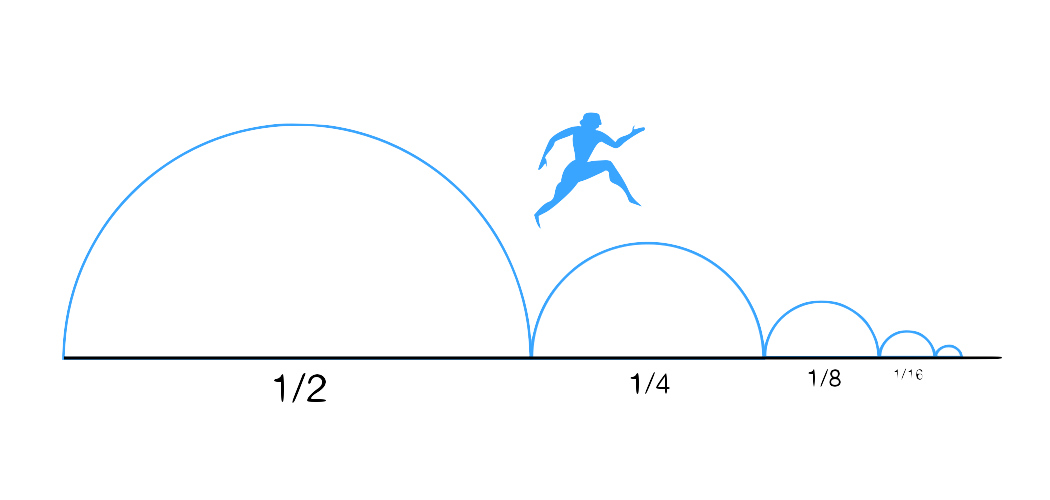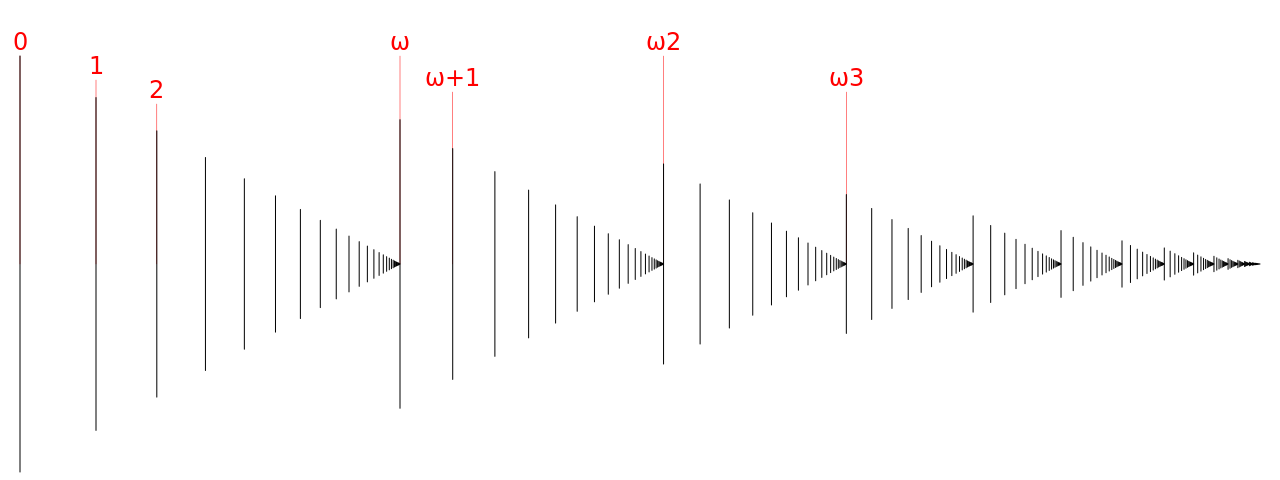# $∞$ Infinity $∞$ ###### Younesse Kaddar

## I. What is it that we call infinity ?

1) Zeno’s Paradoxes 2) Hilbert’s Hotel

## II. How big is it ?

1) Counting to $∞$ : $\aleph_0$ 2) Ordinals : $𝜔$ 3) Inaccessible cardinals

Zeno of Elea : In a race, the quickest runner can never overtake the slowest.![Achilles tries hard](/assets/Slides/Infinity/achilles.jpg) $⇑$ ![Achilles : the explanation](/assets/Slides/Infinity/achilles_explanation.png)Actually, modern math provide a solution :

$$\sum_{n>0} \frac{1}{2^n}$$ is convergent

# Hilbert's Hotel

- Fully occupied - Infinitely many rooms $⟶$ How many additional guests can be housed ?
1) For $n$ new guests : a shift of $n$ 2) How about infintely many new guests ?# II. How big is infinity ?

## Cardinals : $\aleph_0$

Cardinal : the number of elements of an unordered set

$\downarrow$

If the set of all the integers ($ℕ$) exists, we call $\aleph_0$ its cardinal
> **NB** : *Zeno paradox* ⟹ $\aleph_0$ elements can be "written" within a finite space
![aleph_0 elements](/assets/Slides/Infinity/omega.png)

## Ordinals : $𝜔$

Ordinal : the first label you’ll have to use in order to append 1 element to an ordered set

> **NB** : > - *For a finite number of elements* : ordinal ⟺ cardinal > - $\aleph_0 + 1 = \aleph_0$, but $𝜔 + 1 \neq 𝜔$
![omega elements](/assets/Slides/Infinity/omega-0.png)

### Axiom of replacement : to infinity and beyond !

Axiom of replacement : if you take an existing set and replace all elements with something else, you’re left with an other existing set.

We’re going to use it to the fullest !

$$𝜔^{2}$$$𝜔^3$ ![omega_3](/assets/Slides/Infinity/omega-3.png)

<div style=”font-size:400%;” align=center>

$𝜔^4$</div>

<div style=”font-size:400%;” align=center>

$𝜔^𝜔$</div>

<div style=”font-size:400%;” align=center>

$𝜔^{5}$</div>

<div style=”font-size:400%;” align=center>

$𝜔^{𝜔^𝜔}$</div>

<div style=”font-size:400%;” align=center>

$𝜔^{𝜔^{𝜔^{\vdots^{𝜔}}}}$

up to

$𝜀_0$

</div>

# And all that is smaller than …

<div style=”font-size:400%;” align=center>

$\omega_1$ </div>

## corresponding to the cardinal …

<div style=”font-size:400%;” align=center>

$\aleph_1$ </div>

# Then …

<div style=”font-size:300%;” align=center>

$𝜔_2, 𝜔_3, \ldots, 𝜔_{𝜔}, \ldots, 𝜔_{𝜔^{𝜔^{𝜔^{\vdots^{𝜔}}}}}, 𝜔_{𝜀_0}, \ldots$ </div>

## and the corresponding cardinals

<div style=”font-size:400%;” align=center>

$\aleph_2, \aleph_3, \ldots$ </div>

# Power sets : becoming exponentially big

 $𝒫(E) = 2^{ E }$

+

Axiom of replacement

$\downarrow$

# But all of that has an order type … so : it remains smaller than …

<div style=”font-size:400%;” align=center>

Inaccessible cardinals </div>